### Getting started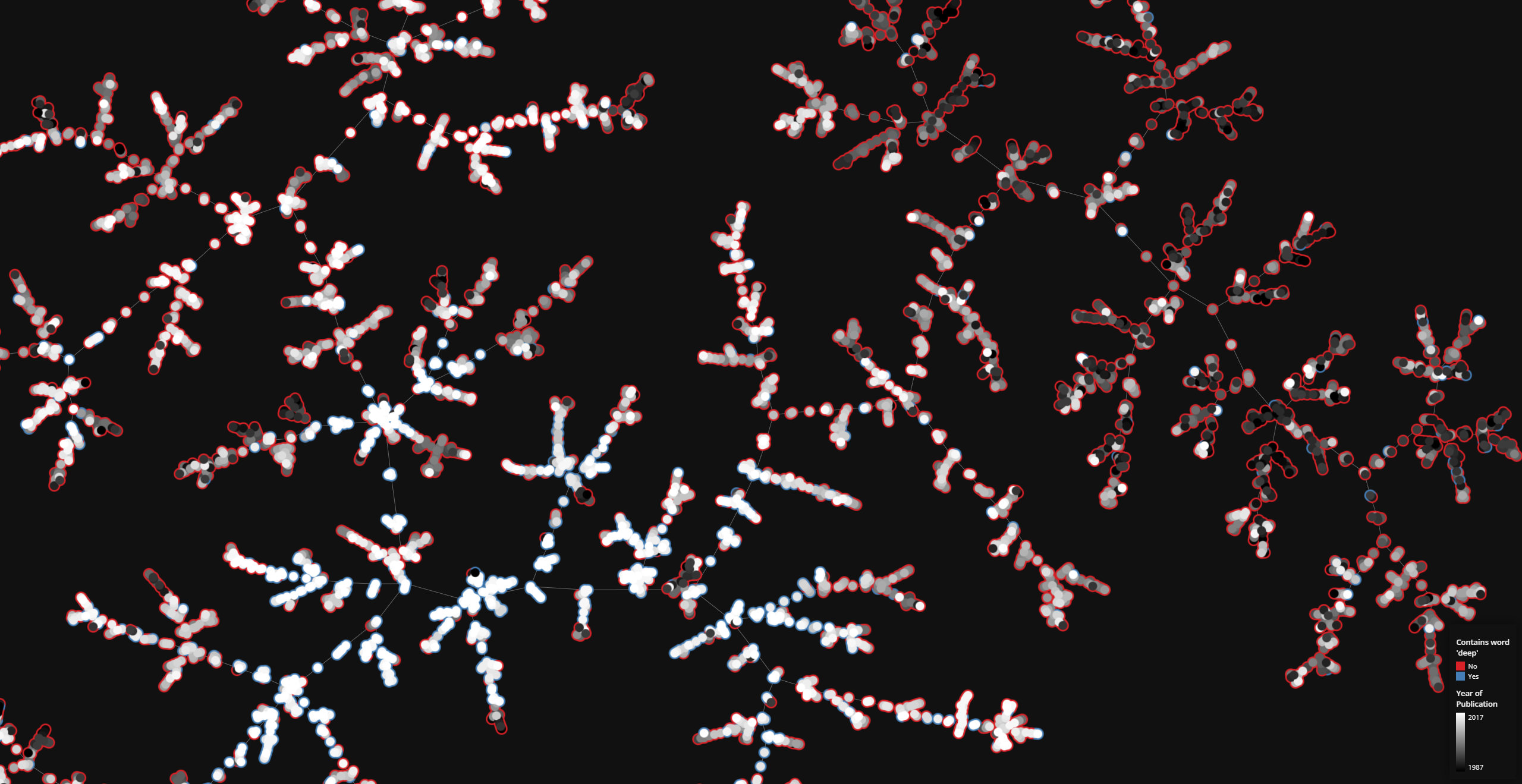tmap is a very fast visualization library for large, high-dimensional data sets. Currently, tmap is available for Python.

NEW: We now provide a web-service that allows for the creaton of TMAP visualizations for small chemical data sets.

#### Supported Environments

Language Operating System Status
Python Linux Available
Windows Available1
macOS Available
R Available2

1Works with WSL
2Through the reticulate R interface to Python.

#### Installation

tmap is installed using the conda package manager. Don't have conda? Download miniconda.

``conda install -c tmap tmap``

We suggest using faerun to plot the data layed out by tmap. But you can of course also use matplotlib (which might be to slow for large data sets and doesn't provide interactive features). Following, an example plot produced with tmap and matplotlib.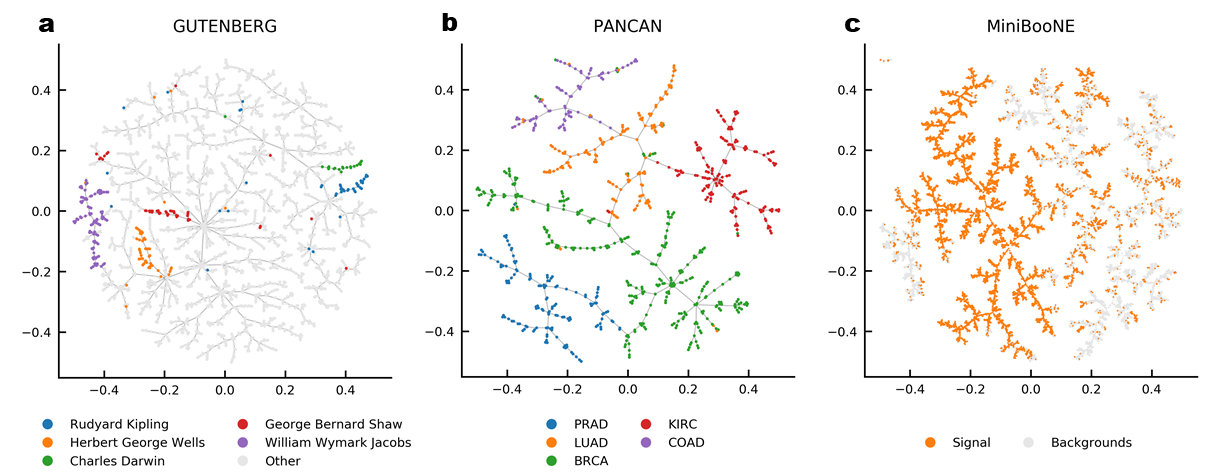``````pip install faerun
# pip install matplotlib``````

#### Laying out a Simple Graph

Even though TMAP is mainly targeted at tasks consisting of laying out very large data sets as trees, the simplest usage example is laying out a graph.

This example produces a matplotlib plot displaying the graph.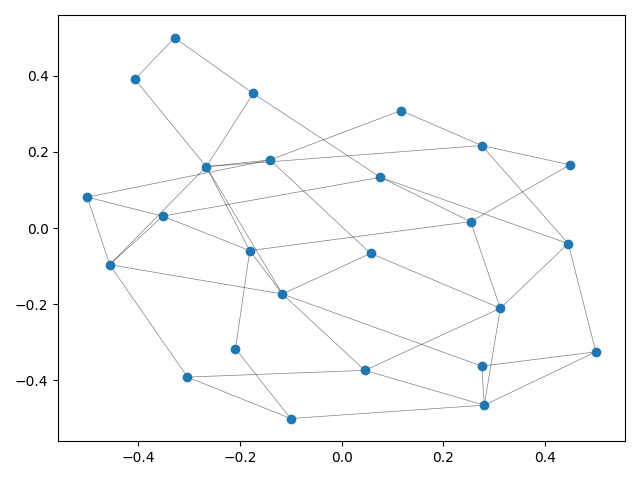When laying out large graphs, it might be useful to discard some edges in order to create a more interpretable and visually pleasing layout. This is achieved using the (default) argument `create_mst=True`. Following, this is exemplified on a small graph.

This example produces a matplotlib plot displaying the graph and it's spanning tree. In the plot on the left, the spanning tree is highlighted by coloring the edges red. The thickness of the edges is inversely related to the edge weight.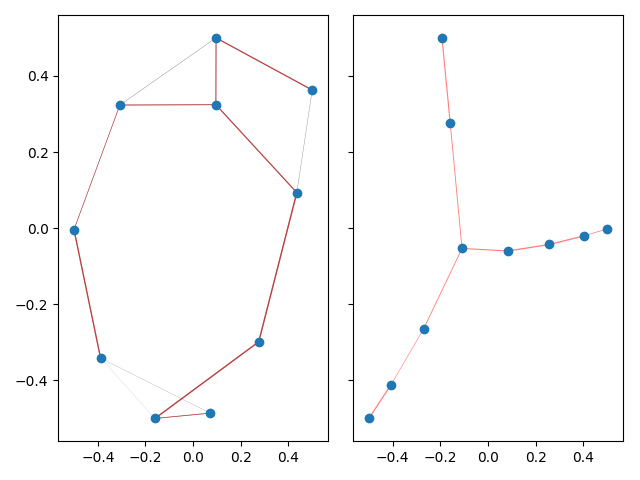On a highly connected graph with 1000 vertices, the advantages of the tree visualizaton method applied by TMAP become obvious.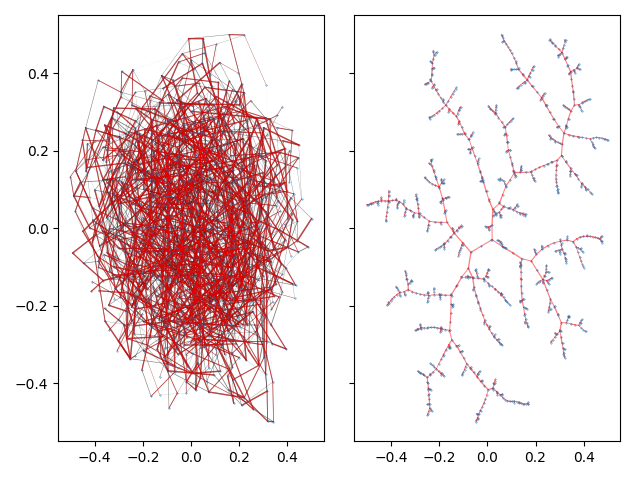There are a wide array of options to tune the final tree layout to your linking. See Layout for the descriptions of all available parameters.

#### MinHash

To enable the visualization of larger data sets, it is necessary to speed up the k-nearest neighbor graph generation. While in general, any approach can be used to create this nearest neighbor graph (see Laying out a Simple Graph), tmap provides a built-in LSH Forest data structure, which enables extremely fast k-nearest neighbor queries.

In order to index data in the LSH forest data structure, it has to be hashed using a locality sensitive scheme such as MinHash.

tmap includes the two classes `Minhash` and `LSHForest` for fast k-nearest neighbor search.

The following example shows how to use the `Minhash` class to estimate Jaccard distances.

``````python minhash.py
0.390625
0.140625``````

#### LSH Forest

The hashes generated by `Minhash` can be indexed using `LSHForest` for fast k-nearest neighbor retreival.

``````python lsh_forest.py
Generating the data took 1164.400289999321ms.
Encoding the data took 155.53330996772274ms.
Indexing took 4.7386749647557735ms.
The kNN search took 0.26249000802636147ms.``````

After indexing the data, the 10 nearest neighbor search on a million 1,000-dimensional vectors took ~0.32ms. In addition, the `LSHForest` class also supports the parallelized generation of a k-nearest neighbor graph using the method `get_knn_graph()`.

``````python lsh_forest_knng.py
Generating the data took 1171.7670540092513ms.
Indexing took 5.959620990324765ms.
The kNN search took 311.5222750348039ms.``````Using matplotlib / pyplot has tow main disadvantages: It is slow and does not yield interactive plots. For this reason, we suggest to use the Python package Faerun for large scale data sets. Faerun supports millions of data points in web-based visualizations.

Together with tmap, Faerun can easily create visualizations of more than 10 million data points including associated web links and structure drawings for high dimensional chemical data sets within an hour.

### Examples

#### COIL 20

Data set dimensions: 1,440×16,384#### MNIST

Data set dimensions: 70,000×764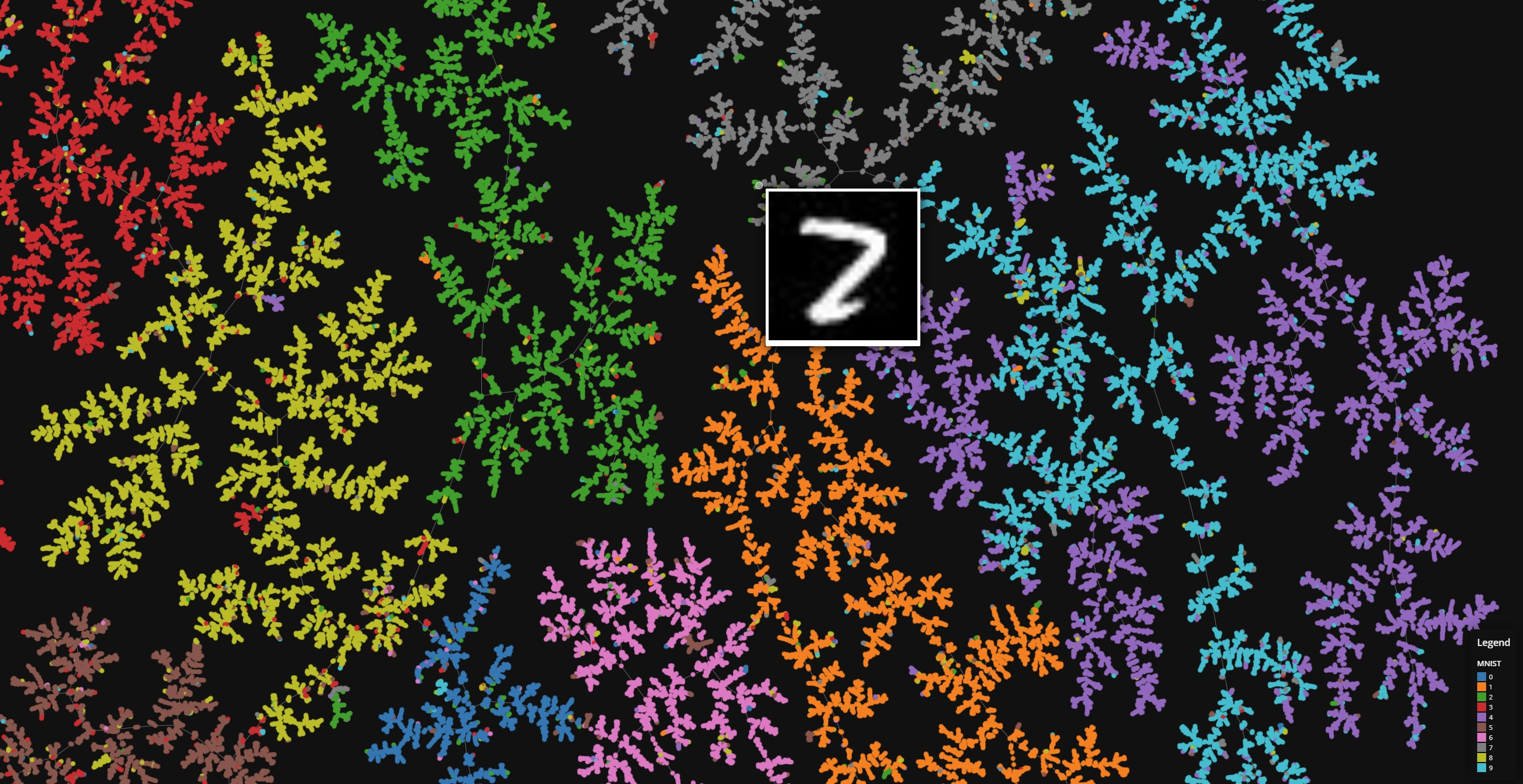#### Fashion MNIST

Data set dimensions: 70,000×764#### ChEMBL

Data set dimensions: 1,159,881×232#### FDB17 and ChEMBL

Data set dimensions: 11,261,085×232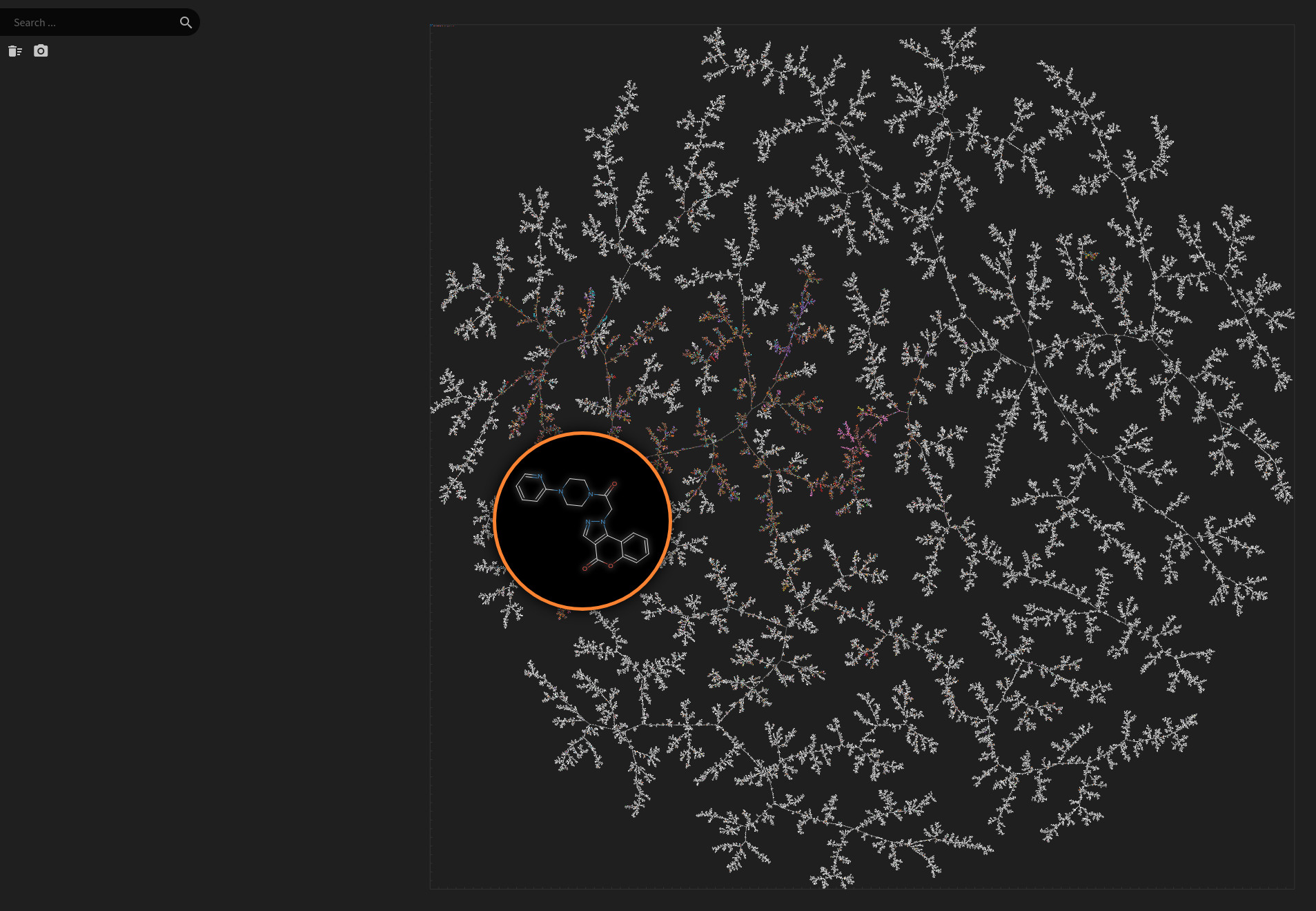#### Natural Product Atlas

Data set dimensions: 24,594×232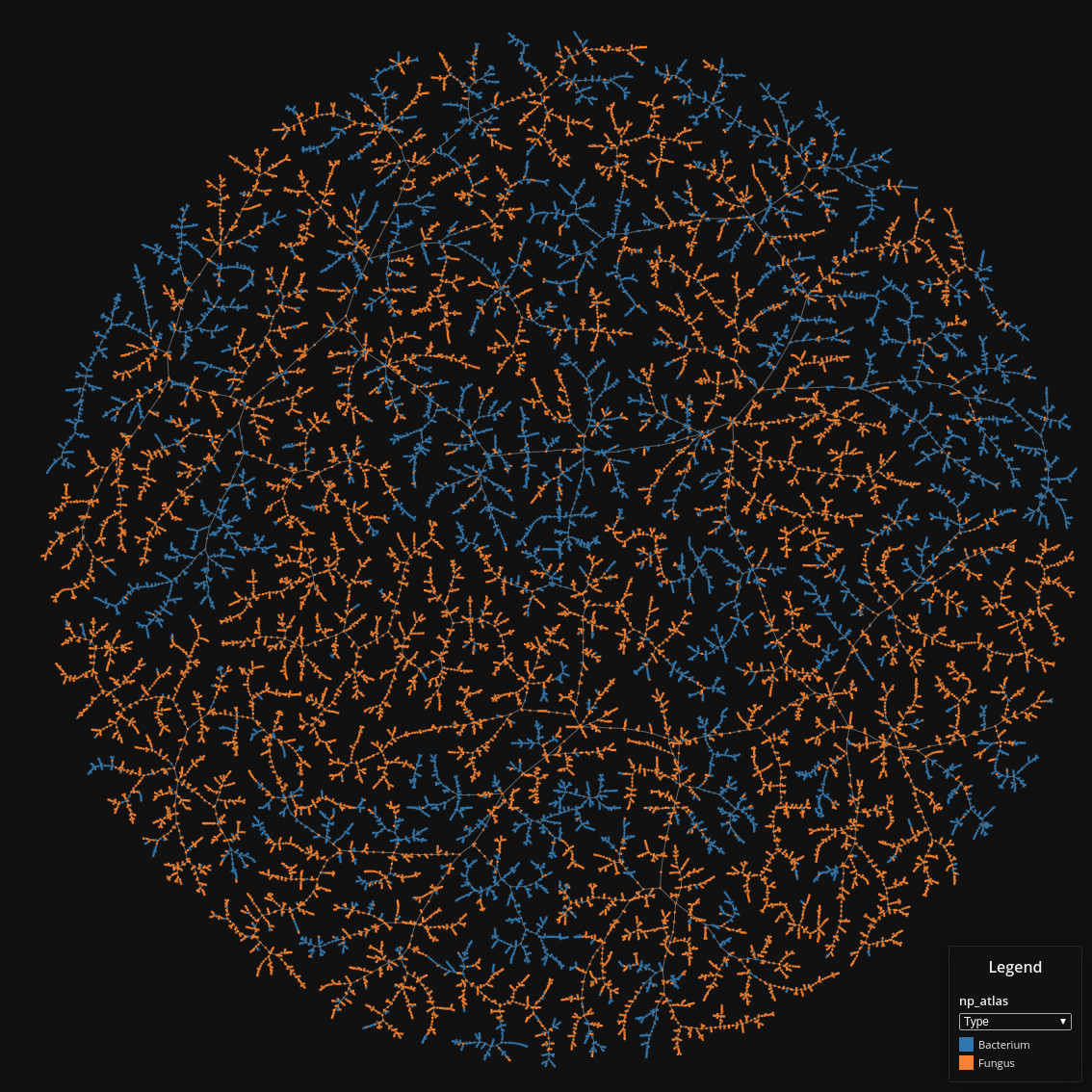#### DSSTox

Data set dimensions: 848,816×232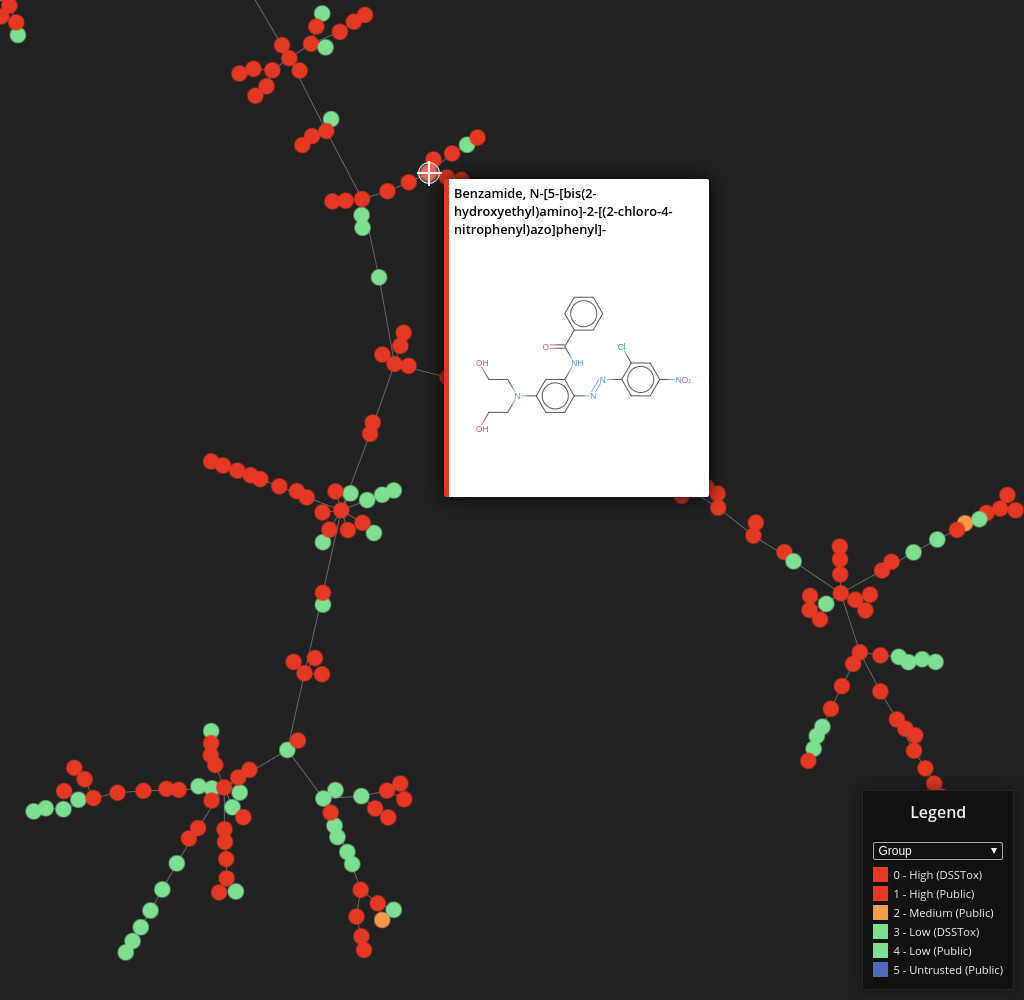#### Protein DataBank

Data set dimensions: 131,236×136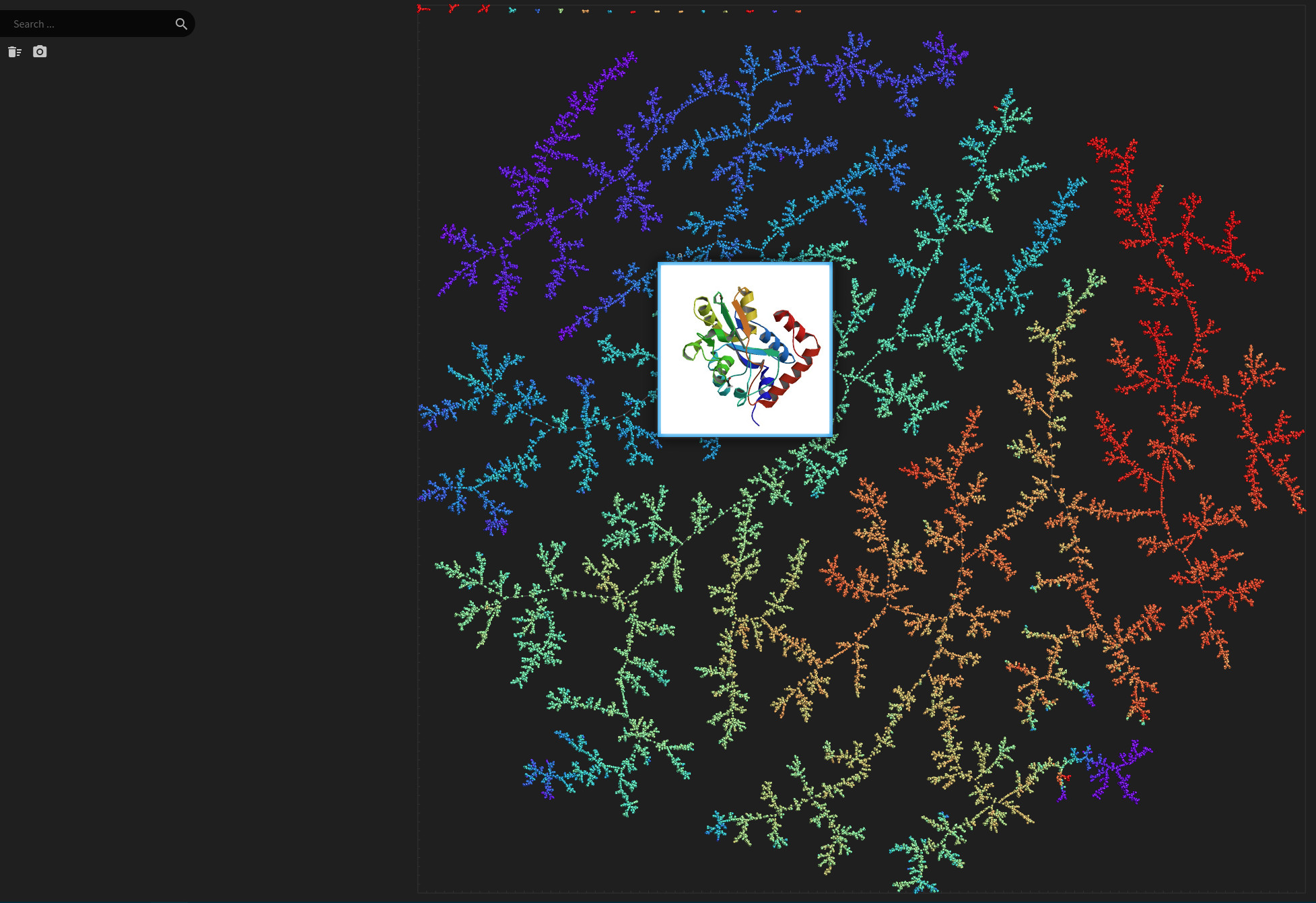#### RNA Sequencing Data

Data set dimensions: 800×1,022#### ProteomeHD

Data set dimensions: 5,013×5,013#### PubMed Central

Data set dimensions: 327,628×1,633,762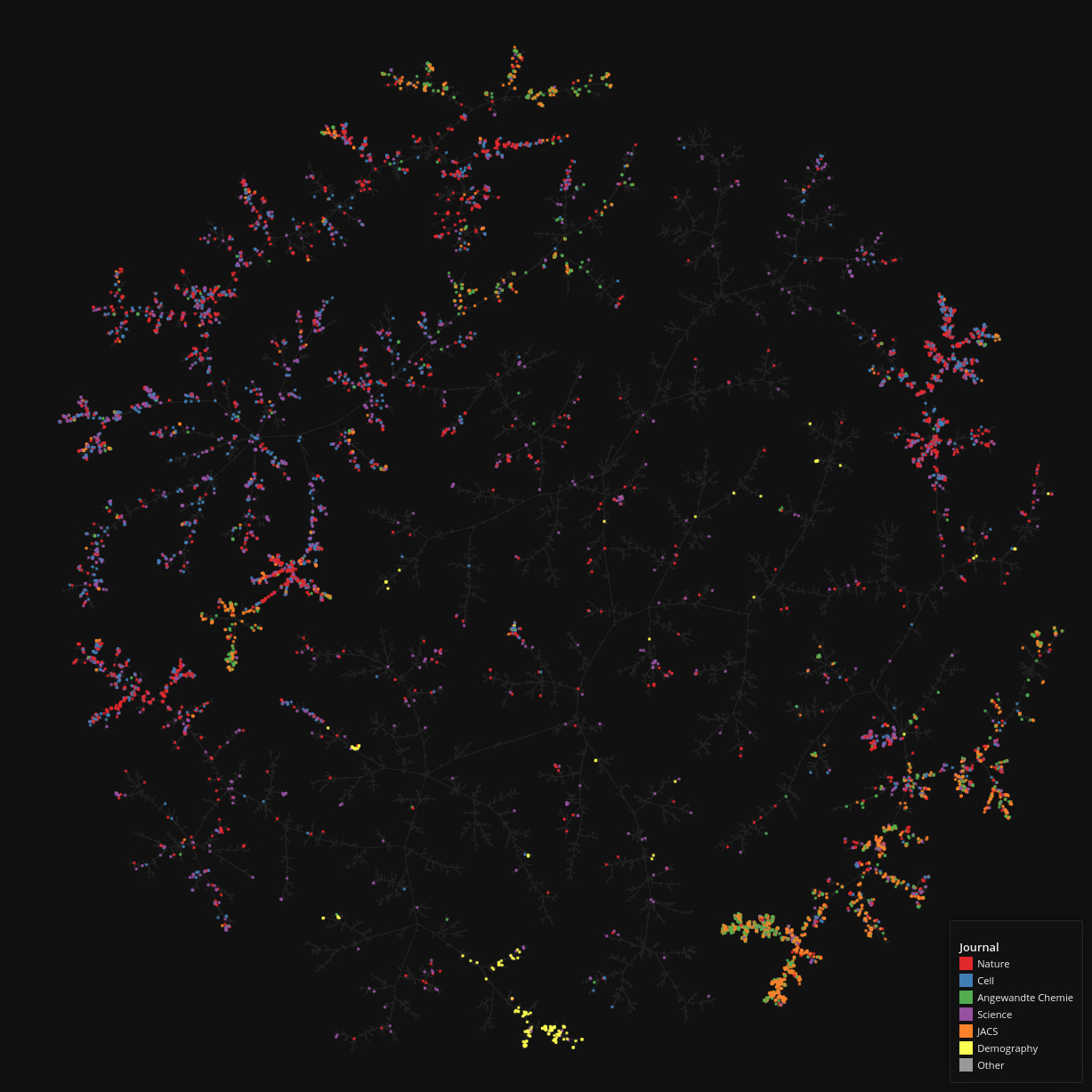#### MiniBooNE

Data set dimensions: 130,065×50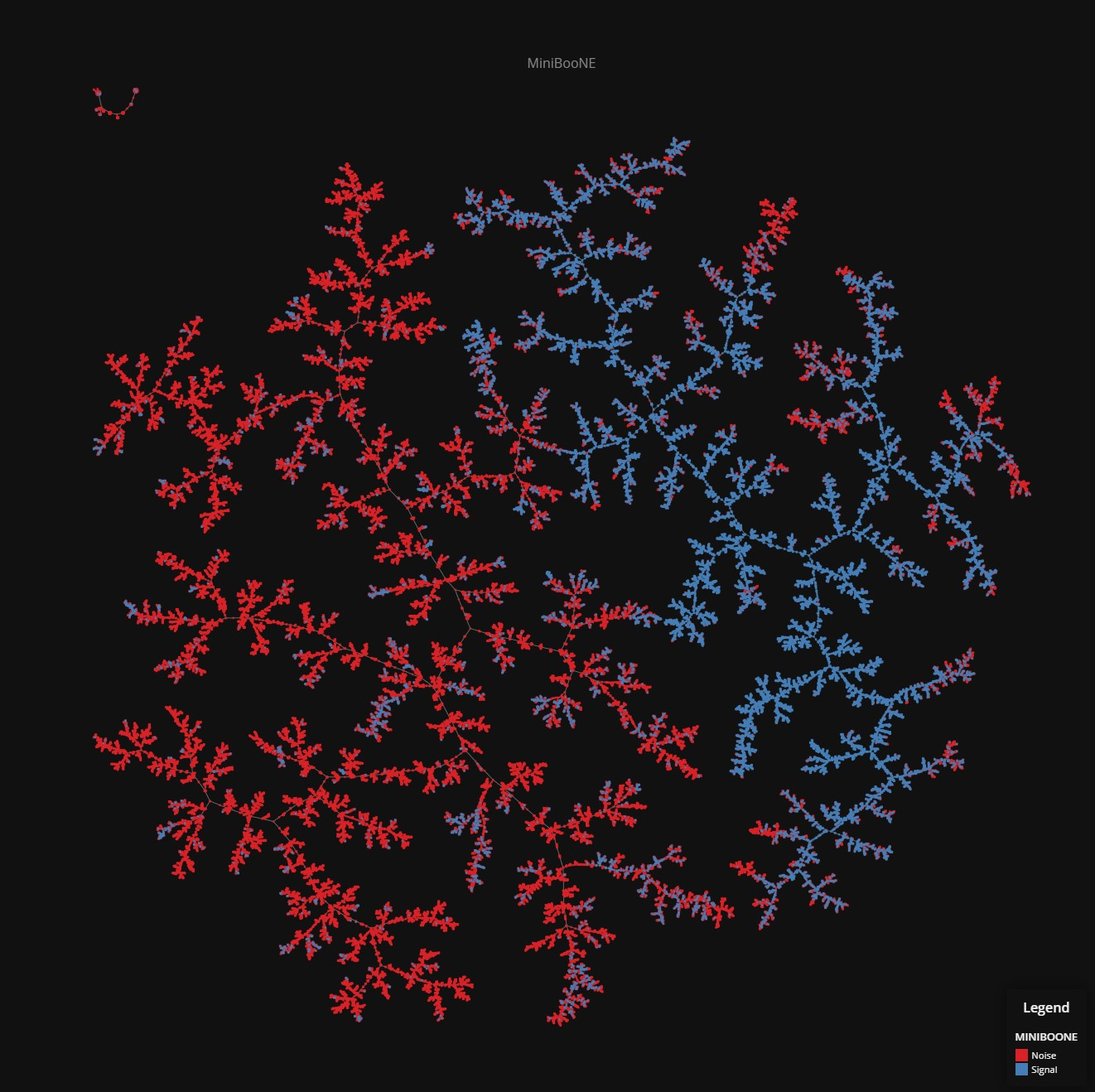#### Gutenberg

Data set dimensions: 3,036×1,217,078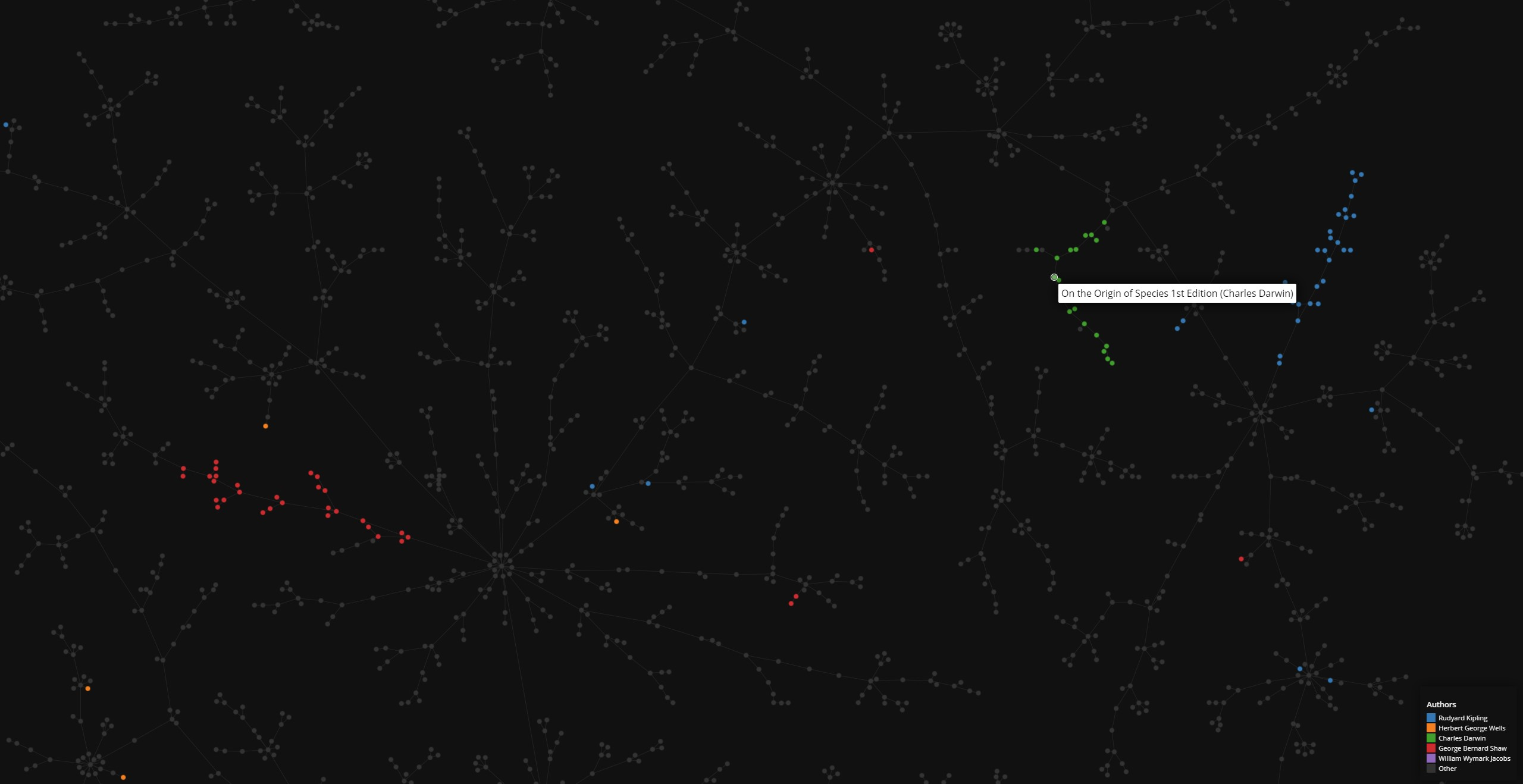#### NIPS Conference Papers

Data set dimensions: 7,241×225,423#### Drugbank

Data set dimensions: 9,300×2^32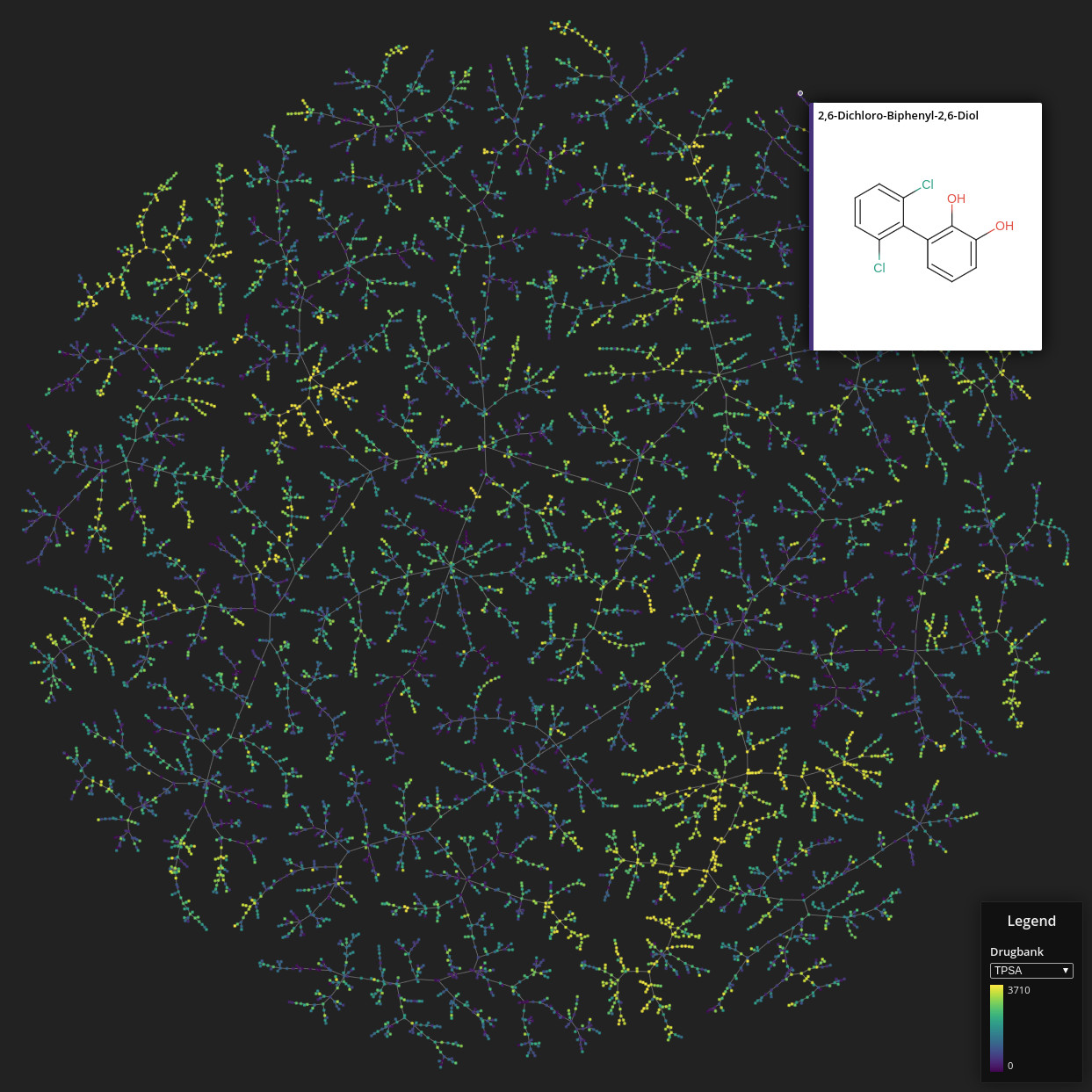#### Flowcytometry

Data set dimensions: 436,877×14### Documentation

#### Minhash

class `tmap.``Minhash`(self: tmap.Minhash, d: int=128, seed: int=42, sample_size: int=128) → None

A generator for MinHash vectors that supports binary, indexed, string and also `int` and `float` weighted vectors as input.

Constructor for the class `Minhash`.

Keyword Arguments
• d (`int`) – The number of permutations used for hashing

• seed (`int`) – The seed used for the random number generator(s)

• sample_size (`int`) – The sample size when generating a weighted MinHash

`batch_from_binary_array`(self: tmap.Minhash, arg0: List[tmap.VectorUchar]) → List[tmap.VectorUint]

Create MinHash vectors from binary arrays (parallelized).

Parameters

vec (`List` of `VectorUchar`) – A list of vectors containing binary values

Returns

A list of MinHash vectors

Return type

`List` of `VectorUint`

`batch_from_int_weight_array`(self: tmap.Minhash, arg0: List[tmap.VectorUint]) → List[tmap.VectorUint]

Create MinHash vectors from `int` arrays, where entries are weights rather than indices of ones (parallelized).

Parameters

vec (`List` of `VectorUint`) – A list of vectors containing `int` values

Returns

A list of MinHash vectors

Return type

`List` of `VectorUint`

`batch_from_sparse_binary_array`(self: tmap.Minhash, arg0: List[tmap.VectorUint]) → List[tmap.VectorUint]

Create MinHash vectors from sparse binary arrays (parallelized).

Parameters

vec (`List` of `VectorUint`) – A list of vectors containing indices of ones in a binary array

Returns

A list of MinHash vectors

Return type

`List` of `VectorUint`

`batch_from_string_array`(self: tmap.Minhash, arg0: List[List[str]]) → List[tmap.VectorUint]

Create MinHash vectors from string arrays (parallelized).

Parameters

vec (`List` of `List` of `str`) – A list of list of strings

Returns

A list of MinHash vectors

Return type

`List` of `VectorUint`

`batch_from_weight_array`(self: tmap.Minhash, vecs: List[tmap.VectorFloat], method: str='ICWS') → List[tmap.VectorUint]

Create MinHash vectors from `float` arrays (parallelized).

Parameters

vec (`List` of `VectorFloat`) – A list of vectors containing `float` values

Keyword Arguments

method (`str`) – The weighted hashing method to use (ICWS or I2CWS)

Returns

A list of MinHash vectors

Return type

`List` of `VectorUint`

`from_binary_array`(self: tmap.Minhash, arg0: tmap.VectorUchar) → tmap.VectorUint

Create a MinHash vector from a binary array.

Parameters

vec (`VectorUchar`) – A vector containing binary values

Returns

A MinHash vector

Return type

`VectorUint`

`from_sparse_binary_array`(self: tmap.Minhash, arg0: tmap.VectorUint) → tmap.VectorUint

Create a MinHash vector from a sparse binary array.

Parameters

vec (`VectorUint`) – A vector containing indices of ones in a binary array

Returns

A MinHash vector

Return type

`VectorUint`

`from_string_array`(self: tmap.Minhash, arg0: List[str]) → tmap.VectorUint

Create a MinHash vector from a string array.

Parameters

vec (`List` of `str`) – A vector containing strings

Returns

A MinHash vector

Return type

`VectorUint`

`from_weight_array`(self: tmap.Minhash, vec: tmap.VectorFloat, method: str='ICWS') → tmap.VectorUint

Create a MinHash vector from a `float` array.

Parameters

vec (`VectorFloat`) – A vector containing `float` values

Keyword Arguments

method (`str`) – The weighted hashing method to use (ICWS or I2CWS)

Returns

A MinHash vector

Return type

`VectorUint`

`get_distance`(self: tmap.Minhash, arg0: tmap.VectorUint, arg1: tmap.VectorUint) → float

Calculate the Jaccard distance between two MinHash vectors.

Parameters
• vec_a (`VectorUint`) – A MinHash vector

• vec_b (`VectorUint`) – A MinHash vector

Returns

`float` The Jaccard distance

`get_weighted_distance`(self: tmap.Minhash, arg0: tmap.VectorUint, arg1: tmap.VectorUint) → float

Calculate the weighted Jaccard distance between two MinHash vectors.

Parameters
• vec_a (`VectorUint`) – A weighted MinHash vector

• vec_b (`VectorUint`) – A weighted MinHash vector

Returns

`float` The Jaccard distance

#### LSHForest

class `tmap.``LSHForest`(self: tmap.LSHForest, d: int=128, l: int=8, store: bool=True, file_backed: bool=False, weighted: bool=False) → None

A LSH forest data structure which incorporates optional linear scan to increase the recovery performance. Most query methods are available in parallelized versions named with a `batch_` prefix.

Constructor for the class `LSHForest`.

Keyword Arguments
• d (`int`) – The dimensionality of the MinHashe vectors to be added

• l (`int`) – The number of prefix trees used when indexing data

• store (`bool`) – Store the data for enhanced querying

• file_backed (`bool`) – Whether to store the data on disk rather than in main memory (experimental)

`add`(self: tmap.LSHForest, arg0: tmap.VectorUint) → None

Add a MinHash vector to the LSH forest.

Parameters

vecs (`VectorUint`) – A MinHash vector that is to be added to the LSH forest

`batch_add`(self: tmap.LSHForest, arg0: List[tmap.VectorUint]) → None

Add a list MinHash vectors to the LSH forest (parallelized).

Parameters

vecs (`List` of `VectorUint`) – A list of MinHash vectors that is to be added to the LSH forest

`batch_query`(self: tmap.LSHForest, arg0: List[tmap.VectorUint], arg1: int) → List[tmap.VectorUint]

Query the LSH forest for k-nearest neighbors (parallelized).

Parameters
• vecs (`List` of `VectorUint`) – The query MinHash vectors

• k (`int`) – The number of nearest neighbors to be retrieved

Returns

The results of the queries

Return type

`List` of `VectorUint`

`clear`(self: tmap.LSHForest) → None

Clears all the added data and computed indices from this `LSHForest` instance.

`get_all_distances`(self: tmap.LSHForest, arg0: tmap.VectorUint) → tmap.VectorFloat

Calculate the Jaccard distances of a MinHash vector to all indexed MinHash vectors.

Parameters

vec (`VectorUint`) – The query MinHash vector

Returns

The Jaccard distances

Return type

`List` of `float`

`get_all_nearest_neighbors`(self: tmap.LSHForest, k: int, kc: int=10) → tmap.VectorUint

Get the k-nearest neighbors of all indexed MinHash vectors.

Parameters

k (`int`) – The number of nearest neighbors to be retrieved

Keyword Arguments

kc (`int`) – The factor by which `k` is multiplied for LSH forest retreival

Returns

`VectorUint` The ids of all k-nearest neighbors

`get_distance`(self: tmap.LSHForest, arg0: tmap.VectorUint, arg1: tmap.VectorUint) → float

Calculate the Jaccard distance between two MinHash vectors.

Parameters
• vec_a (`VectorUint`) – A MinHash vector

• vec_b (`VectorUint`) – A MinHash vector

Returns

`float` The Jaccard distance

`get_distance_by_id`(self: tmap.LSHForest, arg0: int, arg1: int) → float

Calculate the Jaccard distance between two indexed MinHash vectors.

Parameters
• a (`int`) – The id of an indexed MinHash vector

• b (`int`) – The id of an indexed MinHash vector

Returns

`float` The Jaccard distance

`get_hash`(self: tmap.LSHForest, arg0: int) → tmap.VectorUint

Retrieve the MinHash vector of an indexed entry given its index. The index is defined by order of insertion.

Parameters

a (`int`) – The id of an indexed MinHash vector

Returns

`VectorUint` The MinHash vector

`get_knn_graph`(self: tmap.LSHForest, from: tmap.VectorUint, to: tmap.VectorUint, weight: tmap.VectorFloat, k: int, kc: int=10) → None

Construct the k-nearest neighbor graph of the indexed MinHash vectors. It will be written to out parameters `from`, `to`, and `weight` as an edge list.

Parameters
• from (`VectorUint`) – A vector to which the ids for the from vertices are written

• to (`VectorUint`) – A vector to which the ids for the to vertices are written

• weight (`VectorFloat`) – A vector to which the edge weights are written

• k (`int`) – The number of nearest neighbors to be retrieved during the construction of the k-nearest neighbor graph

Keyword Arguments

kc (`int`) – The factor by which `k` is multiplied for LSH forest retreival

`get_weighted_distance`(self: tmap.LSHForest, arg0: tmap.VectorUint, arg1: tmap.VectorUint) → float

Calculate the weighted Jaccard distance between two MinHash vectors.

Parameters
• vec_a (`VectorUint`) – A weighted MinHash vector

• vec_b (`VectorUint`) – A weighted MinHash vector

Returns

`float` The Jaccard distance

`get_weighted_distance_by_id`(self: tmap.LSHForest, arg0: int, arg1: int) → float

Calculate the Jaccard distance between two indexed weighted MinHash vectors.

Parameters
• a (`int`) – The id of an indexed weighted MinHash vector

• b (`int`) – The id of an indexed weighted MinHash vector

Returns

`float` The weighted Jaccard distance

`index`(self: tmap.LSHForest) → None

Index the LSH forest. This has to be run after each time new MinHashes were added.

`is_clean`(self: tmap.LSHForest) → bool

Returns a boolean indicating whether or not the LSH forest has been indexed after the last MinHash vector was added.

Returns

`True` if `index()` has been run since MinHash vectors have last been added using `add()` or `batch_add()`. `False` otherwise

Return type

`bool`

`linear_scan`(self: tmap.LSHForest, vec: tmap.VectorUint, indices: tmap.VectorUint, k: int=10) → List[Tuple[float, int]]

Query a subset of indexed MinHash vectors using linear scan.

Parameters
• vec (`VectorUint`) – The query MinHash vector

• indices (`VectorUint`) –

Keyword Arguments

k (`int`) – The number of nearest neighbors to be retrieved

Returns

The results of the query

Return type

`List` of ```Tuple[float, int]```

`query`(self: tmap.LSHForest, arg0: tmap.VectorUint, arg1: int) → tmap.VectorUint

Query the LSH forest for k-nearest neighbors.

Parameters
• vec (`VectorUint`) – The query MinHash vector

• k (`int`) – The number of nearest neighbors to be retrieved

Returns

The results of the query

Return type

`VectorUint`

`query_by_id`(self: tmap.LSHForest, arg0: int, arg1: int) → tmap.VectorUint

Query the LSH forest for k-nearest neighbors.

Parameters
• id (`int`) – The id of an indexed MinHash vector

• k (`int`) – The number of nearest neighbors to be retrieved

Returns

The results of the query

Return type

`VectorUint`

`query_exclude`(self: tmap.LSHForest, arg0: tmap.VectorUint, arg1: tmap.VectorUint, arg2: int) → tmap.VectorUint

Query the LSH forest for k-nearest neighbors.

Parameters
• vec (`VectorUint`) – The query MinHash vector

• exclude (`List` of `VectorUint`) –

• k (`int`) – The number of nearest neighbors to be retrieved

Returns

The results of the query

Return type

`VectorUint`

`query_exclude_by_id`(self: tmap.LSHForest, arg0: int, arg1: tmap.VectorUint, arg2: int) → tmap.VectorUint

Query the LSH forest for k-nearest neighbors.

Parameters
• id (`int`) – The id of an indexed MinHash vector

• exclude (`List` of `VectorUint`) –

• k (`int`) – The number of nearest neighbors to be retrieved

Returns

The results of the query

Return type

`VectorUint`

`query_linear_scan`(self: tmap.LSHForest, vec: tmap.VectorUint, k: int, kc: int=10) → List[Tuple[float, int]]

Query k-nearest neighbors with a LSH forest / linear scan combination. `k`*:obj:`kc` nearest neighbors are searched for using LSH forest; from these, the `k` nearest neighbors are retrieved using linear scan.

Parameters
• vec (`VectorUint`) – The query MinHash vector

• k (`int`) – The number of nearest neighbors to be retrieved

Keyword Arguments

kc (`int`) – The factor by which `k` is multiplied for LSH forest retreival

Returns

The results of the query

Return type

`List` of ```Tuple[float, int]```

`query_linear_scan_by_id`(self: tmap.LSHForest, id: int, k: int, kc: int=10) → List[Tuple[float, int]]

Query k-nearest neighbors with a LSH forest / linear scan combination. `k`*:obj:`kc` nearest neighbors are searched for using LSH forest; from these, the `k` nearest neighbors are retrieved using linear scan.

Parameters
• id (`int`) – The id of an indexed MinHash vector

• k (`int`) – The number of nearest neighbors to be retrieved

Keyword Arguments

kc (`int`) – The factor by which `k` is multiplied for LSH forest retreival

Returns

The results of the query

Return type

`List` of ```Tuple[float, int]```

`query_linear_scan_exclude`(self: tmap.LSHForest, vec: tmap.VectorUint, k: int, exclude: tmap.VectorUint, kc: int=10) → List[Tuple[float, int]]

Query k-nearest neighbors with a LSH forest / linear scan combination. `k`*:obj:`kc` nearest neighbors are searched for using LSH forest; from these, the `k` nearest neighbors are retrieved using linear scan.

Parameters
• vec (`VectorUint`) – The query MinHash vector

• k (`int`) – The number of nearest neighbors to be retrieved

Keyword Arguments
• exclude (`List` of `VectorUint`) –

• kc (`int`) – The factor by which `k` is multiplied for LSH forest retreival

Returns

The results of the query

Return type

`List` of ```Tuple[float, int]```

`query_linear_scan_exclude_by_id`(self: tmap.LSHForest, id: int, k: int, exclude: tmap.VectorUint, kc: int=10) → List[Tuple[float, int]]

Query k-nearest neighbors with a LSH forest / linear scan combination. `k`*:obj:`kc` nearest neighbors are searched for using LSH forest; from these, the `k` nearest neighbors are retrieved using linear scan.

Parameters
• id (`int`) – The id of an indexed MinHash vector

• k (`int`) – The number of nearest neighbors to be retrieved

Keyword Arguments
• exclude (`List` of `VectorUint`) –

• kc (`int`) – The factor by which `k` is multiplied for LSH forest retreival

Returns

The results of the query

Return type

`List` of ```Tuple[float, int]```

`restore`(self: tmap.LSHForest, arg0: str) → None

Deserializes a previously serialized (using `store()`) state into this instance of `LSHForest` and recreates the index.

Parameters

path (`str`) – The path to the file which is deserialized

`size`(self: tmap.LSHForest) → int

Returns the number of MinHash vectors in this LSHForest instance.

Returns

The number of MinHash vectors

Return type

`int`

`store`(self: tmap.LSHForest, arg0: str) → None

Serializes the current state of this instance of `LSHForest` to the disk in binary format. The index is not serialized and has to be rebuilt after deserialization.

Parameters

path (`str`) – The path to which to searialize the file

#### Layout

layout_from_lsh_forest(lsh_forest: tmap::LSHForest, config: tmap.LayoutConfiguration=tmap.LayoutConfiguration(), create_mst: bool=True, clear_lsh_forest: bool=False, weighted: bool=False) → Tuple[tmap.VectorFloat, tmap.VectorFloat, tmap.VectorUint, tmap.VectorUint, tmap.GraphProperties]

Create minimum spanning tree or k-nearest neighbor graph coordinates and topology from an `LSHForest` instance.

Parameters

lsh_forest (`LSHForest`) – An `LSHForest` instance

Keyword Arguments
Returns

The x and y coordinates of the vertices, the ids of the vertices spanning the edges, and information on the graph

Return type
```Tuple[VectorFloat, VectorFloat, VectorUint, VectorUint, GraphProperties]```
layout_from_edge_list(vertex_count: int, edges: List[Tuple[int, int, float]], config: tmap.LayoutConfiguration=tmap.LayoutConfiguration(), create_mst: bool=True) → Tuple[tmap.VectorFloat, tmap.VectorFloat, tmap.VectorUint, tmap.VectorUint, tmap.GraphProperties]

Create minimum spanning tree or k-nearest neighbor graph coordinates and topology from an edge list.

Parameters

vertex_count (`int`): The number of vertices in the edge list edges (`List` of ```Tuple[int, int, float]```) – An edge list defining a graph

Keyword Arguments:
Returns

The x and y coordinates of the vertices, the ids of the vertices spanning the edges, and information on the graph

Return type
```Tuple[VectorFloat, VectorFloat, VectorUint, VectorUint, GraphProperties]```
`tmap.``mst_from_lsh_forest`(lsh_forest: tmap::LSHForest, k: int, kc: int=10, weighted: bool=False) → Tuple[tmap.VectorUint, tmap.VectorUint]

Create minimum spanning tree topology from an `LSHForest` instance.

Parameters
Keyword Arguments
• kc (int) – The scalar by which k is multiplied before querying the LSH forest. The results are then ordered decreasing based on linear-scan distances and the top k results returned

• weighted (`bool`) – Whether the MinHash vectors in the `LSHForest` instance are weighted

Returns

the topology of the minimum spanning tree of the data indexed in the LSH forest

Return type

```Tuple[VectorUint, VectorUint]```

class `tmap.``ScalingType`(self: tmap.ScalingType, arg0: int) → None

The scaling types available in OGDF. The class is to be used as an enum.

Notes

The available values are

`ScalingType.Absolute`: Absolute factor, can be used to scale relative to level size change.

`ScalingType.RelativeToAvgLength`: Scales by a factor relative to the average edge weights.

`ScalingType.RelativeToDesiredLength`: Scales by a factor relative to the disired edge length.

`ScalingType.RelativeToDrawing`: Scales by a factor relative to the drawing.

class `tmap.``Placer`(self: tmap.Placer, arg0: int) → None

The places available in OGDF. The class is to be used as an enum.

Notes

The available values are

`Placer.Barycenter`: Places a vertex at the barycenter of its neighbors’ position.

`Placer.Solar`: Uses information of the merging phase of the solar merger. Places a new vertex on the direct line between two suns.

`Placer.Circle`: Places the vertices in a circle around the barycenter and outside of the current drawing

`Placer.Median`: Places a vertex at the median position of the neighbor nodes for each coordinate axis.

`Placer.Random`: Places a vertex at a random position within the smallest circle containing all vertices around the barycenter of the current drawing.

`Placer.Zero`: Places a vertex at the same position as its representative in the previous level.

class `tmap.``Merger`(self: tmap.Merger, arg0: int) → None

The mergers available in OGDF. The class is to be used as an enum.

Notes

The available values are

`Merger.EdgeCover`: Based on the matching merger. Computes an edge cover such that each contained edge is incident to at least one unmatched vertex. The cover edges are then used to merge their adjacent vertices.

`Merger.LocalBiconnected`: Based on the edge cover merger. Avoids distortions by checking whether biconnectivity will be lost in the local neighborhood around the potential merging position.

`Merger.Solar`: Vertices are partitioned into solar systems, consisting of sun, planets and moons. The systems are then merged into the sun vertices.

`Merger.IndependentSet`: Uses a maximal independent set filtration. See GRIP for details.

class `tmap.``LayoutConfiguration`(self: tmap.LayoutConfiguration) → None

A container for configuration options for `layout_from_lsh_forest()` and `layout_from_edge_list()`.

`int k`

The number of nearest neighbors used to create the k-nearest neighbor graph.

Type

`int`

`int kc`

The scalar by which k is multiplied before querying the LSH forest. The results are then ordered decreasing based on linear-scan distances and the top k results returned.

Type

`int`

`int fme_iterations`

Maximum number of iterations of the fast multipole embedder.

Type

`int`

`bool fme_randomize`

Whether or not to randomize the layout at the start.

Type

`bool`

`int fme_threads`

The number of threads for the fast multipole embedder.

Type

`int`

`int fme_precision`

The number of coefficients of the multipole expansion.

Type

`int`

`int sl_repeats`

The number of repeats of the scaling layout algorithm.

Type

`int`

`int sl_extra_scaling_steps`

Sets the number of repeats of the scaling.

Type

`int`

`double sl_scaling_min`

The minimum scaling factor.

Type

`float`

`double sl_scaling_max`

The maximum scaling factor.

Type

`float`

`ScalingType sl_scaling_type`

Defines the (relative) scale of the graph.

Type
`int mmm_repeats`

Number of repeats of the per-level layout algorithm.

Type

`int`

`Placer placer`

The method by which the initial positons of the vertices at eachlevel are defined.

Type
`Merger merger`

The vertex merging strategy applied during the coarsening phaseof the multilevel algorithm.

Type
`double merger_factor`

The ratio of the sizes between two levels up to which the mergingis run. Does not apply to all merging strategies.

Type

`float`

`int merger_adjustment`

The edge length adjustment of the merging algorithm. Does notapply to all merging strategies.

Type

`int`

`float node_size`

The size of the nodes, which affects the magnitude of their repellingforce. Decreasing this value generally resolves overlaps in a verycrowded tree.

Type

`float`

Constructor for the class `LayoutConfiguration`.

`__init__`(self: tmap.LayoutConfiguration) → None

Constructor for the class `LayoutConfiguration`.

class `tmap.``GraphProperties`(self: tmap.GraphProperties) → None

Contains properties of the minimum spanning tree (or forest) generated by `layout_from_lsh_forest()` and `layout_from_edge_list()`.

`mst_weight`

The total weight of the minimum spanning tree.

Type

`float`

`n_connected_components`

The number of connected components in the minimum spanning forest.

Type

`int`

`n_isolated_vertices`
Type

`int`

`degrees`

The degrees of all vertices in the minimum spanning tree (or forest).

Type

`VectorUint`

`adjacency_list`

The adjaceny lists for all vertices in the minimum spanning tree (or forest).

Type

`List` of `VectorUint`

Constructor for the class `GraphProperties`.

`__init__`(self: tmap.GraphProperties) → None

Constructor for the class `GraphProperties`.